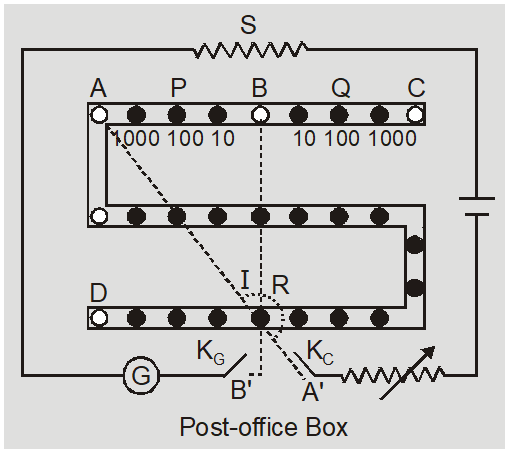# Post Office Box Physics || Current Electricity Class 12, JEE & NEET

A Post Office Box can also be used to measure an unknown resistance. It is a Wheatstone Bridge with three arms P, Q and R; while the fourth arm(s) is the unknown resistance. P and Q are known as the ratio arms while R is known as the rheostat arm. Post Office Box, Physics named it so because it has a shape of box and was designed to find resistance of electric cables and telegraph wires. It was used in post offices to determine resistance of transmission lines.
1. At balance, the unknown resistance S = (P/Q) R                                                           …… (1)
2. It is based on principle of Wheatstone bridge.
3. Unknown resistance is $\mathrm{S}=\frac{\mathrm{Q}}{\mathrm{P}} \mathrm{R}$ and specific resistance is $\rho=\frac{\pi r^{2} S}{L},$ where $r$ is radius and $L$ is length of wire.
4. In Post Office box we first press cell key and then press galvanometer key to eliminate induced effects.5. It is used to find unknown resistance, specific resistance of a wire, internal resistance of cell, resistance of galvanometer etc.
6. A typical post office box is in a wooden box with a hinged lid and a metal or Bakelite panel showing circuit connections. Coils of wire are wound non-inductively, mounted in the body of the box, and have a negligible temperature coefficient. Pairs of ratio arms are each 10, 100, 1000 ohms. A resistance arm contains a number of coils from 1 to 5000 ohms with a plug for infinite resistance.
Physics Revision Series by Saransh Sir (AIR 41 in IIT-JEE) Also Read: